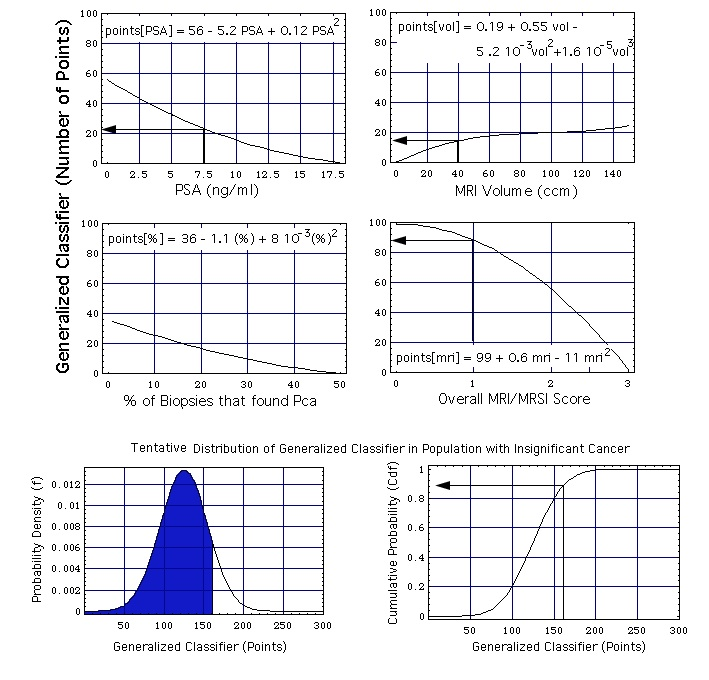## Generation and Evaluation of a Generalized Classifier for Prostate Cancer

Data and Method from
Shukla-Dave A, Hricak H, Kattan MW, Pucar D, Kuroiwa K, Chen HN, Spector J, Koutcher JA, Zakian KL, Scardino PT
The utility of magnetic resonance imaging and spectroscopy for predicting insignificant prostate cancer: an initial analysis.
BJU Int. 99(4):786-793 (April 2007)

Since single parameters ("variables"), such as

1. total PSA concentration,
2. prostate volume,
3. biopsies or
4. sophisticated MRI/MRSI
do not provide sufficient sensitivity and specificity (e.g. in predicting the insignificance of prostate cancers), models have been constructed that combine such single variables. The model parameters are
1. the weights with which each single variable is chosen to contribute to the lumped parameter (henceforth called "generalized classifier") and
2. the distribution of the lumped parameter over the investigated population.
The parameters are fitted such that the clinical findings are best reproduced.

A particularly transparent model representation has been published by the above mentioned authors in the form of a nomogram. Here a mathematically identical alternative is presented: Instead of a nomogram curves specify how much each single variable contributes to the generalized classifier.

### Construction of the Model

In the first step, the weights are defined with which the 4 single variables enter into the generalized classifier:
• Every curve in the upper 4 diagrams of the figure below specifies the weight (expressed as a number of points). For example, a PSA concentration 7.5 ng/ml is assigned a weight of 23 points: points[PSA = 7.5 ng/ml] = 23.
• The analytic form of every curve is the Taylor series given in the top part of the diagram.
• The numerical coefficients in these Taylor series are fitted in a calibration process described below.Figure: The MRI/MRSI model, combining the single parameters PSA concentration, prostate volume, percentage of positive biopsies, and overall MRI/MRSI score. The two probability functions at the bottom of the figure are the ones defined earlier: probability density function f = f[x, mu, sig] and the cumulative probability function Cdf = Cdf[x, mu, sig] with mu = 125 and sig = 30.

Example of how -with the help of this figure- a generalized classifier point value x is calculated from the set of 4 single variables:

1. A (total) PSA concentration of 7.5 ng/mL contributes 23 points to the generalized classifier,
2. a prostate volume of 40 cm3 contributes 15 points, and
3. when no biopsy speciment shows cancer this amounts to 35 points,

which adds up to 73 points.

4. When the MRSI has as result "probably insignificant" (overall MRI/MRSI score = 1), this amounts to an additional contribution of 88 points to the generalized classifier.
The total classifier point number in the given example is (73 + 88 + 0) points = 161 points. As one can see, the MRI/MRSI overall score is given the largest weight (88 points).

The second step of the assessment (lower 2 diagrams of figure) is as empirical as the first one: It is assumed that the classifier is normally distributed. The lower left hand part of figure shows the probability density f[x, mu, sig]. mu is the position of the maximum of f[x, mu, sig], sig is the width of f[x, mu, sig]. The lower right hand part of the figure shows the cumulative probability Cdf[x, mu, sig]. In the chosen example

• the area under f[x, mu, sig] and left of a vertical at x = 161 points (blue, lower left hand corner of figure) is the computed ("predicted") cumulative probability that a person with a generalized classifier value x = 161 has insignificant cancer (i.e. 86 %, see the cumulative distribution function, lower right hand corner).
• The actual cumulative probability for a given classifier point value x is the (in surgical pathology) observed fraction of insignificant cases that has a classifier point value smaller than or equal to x.

### Calibration of the Model

The model is calibrated by adjusting
• the numerical coefficients in the 4 equations points[PSA], points[vol], points[%], points[mri], and
• the location mu and width sig of the probabilty functions f and Cdf.
The coefficients are fitted such that for all sums

generalized classifier = points[PSA] + points[vol] + points[%] + points[mri]

the predicted probabilities come as close as possible to the actually observed probabilities. This multiparameter fit process does not produce unique coefficients, meaning that e.g. several sets of Taylor coefficients are similarly well suited to reproduce the observed cancer probabilities.

### Validation of the Model

The model is validated with the "leave-one-out" (also called "jack-knife") cross validation: the data set of all n patients (A, B, C, ... Z) is divided in two parts:
1. the data associated with n-1 patients (B, C, ...Z),
2. the data associated with the remaining single patient A ("validation patient"),
The data associated with the n-1 patients are used for calibration, i.e. for fitting the Taylor series coefficients and the probability curve parameters (mu and sig). The data associated with patient A are used for validation.

This process is repeated n consecutive times, each time with a different validation patient.

back to the MRI/MRSI model.

version: August 18, 2012
address of this page
home
Joachim Gruber